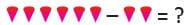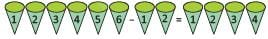## 10 Questions MCQ Test Math Olympiad for Class 2 | Olympiad Test Level 1: Addition and Subtraction - 1

Description
Attempt Olympiad Test Level 1: Addition and Subtraction - 1 | 10 questions in 20 minutes | Mock test for Class 2 preparation | Free important questions MCQ to study Math Olympiad for Class 2 for Class 2 Exam | Download free PDF with solutions
QUESTION: 1

### Sonali had Rs.40 in her piggy bank. She went to a shop to purchase a Notebook of Rs. 55. How much less amount Sonali had, to purchase the notebook.

Solution:

Less amount = Rs. 55 – Rs. 40
= Rs.15

QUESTION: 2

Solution:

90 – 30 = 60

QUESTION: 3

### Direction: Find the missing number. 43 – ____ = 24

Solution:

43 – 19 = 24

QUESTION: 4

Aviral has 7 blue balls, 8 red balls and 6 black balls. How many balls Aviral has ?

Solution:

Total balls = 7 + 8 + 6 = 21 balls

QUESTION: 5

Rishi had 43 oranges. Out of them, 13 oranges were rotten. How many fresh oranges Rishi has ?

Solution:

43 – 13 = 30 fresh oranges.

QUESTION: 6

There are 15 novels and 16 biographies in a rack. How many total Novels and Biographies are present in the rack ?

Solution:

15 Novels + 16 Biographies = 31

QUESTION: 7

Find the missing number.
81 – ________ = 73

Solution:

81 – 8 = 73

QUESTION: 8

Find the missing number.
5 + _______ = 13

Solution:

5 + 8 = 13

QUESTION: 9Solution:QUESTION: 10

7 + 9 + 1 + 4 = ?

Solution:

4 + 9 + 1 + 7 = 21Use Code STAYHOME200 and get INR 200 additional OFF Use Coupon Code